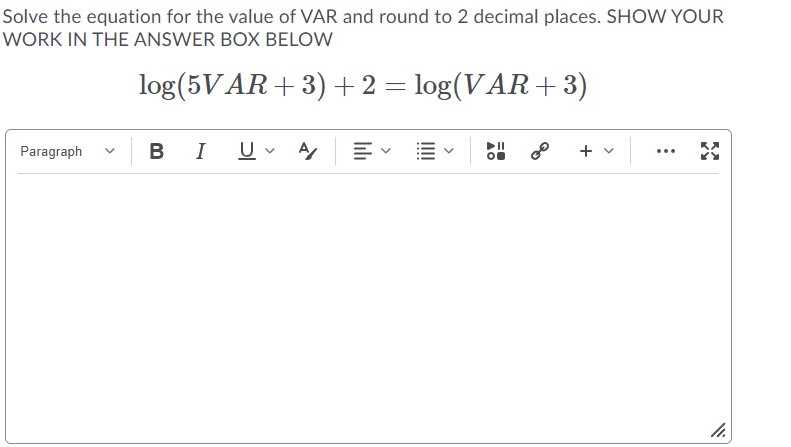### Still have math questions?

Algebra
QuestionSolve the equation for the value of VAR and round to $$2$$ decimal places. SHOW YOUR WORK IN THE ANSWER BOX BELOW

$$\log ( 5 V A R + 3 ) + 2 = \log ( V A R + 3 )$$

$$\log(500VAR+ 300)= \log (VAR + 3)\\500VAR+ 300= VAR+ 3\\499VAR= - 297\\VAR= - \frac{297}{499} \approx - 0.60$$